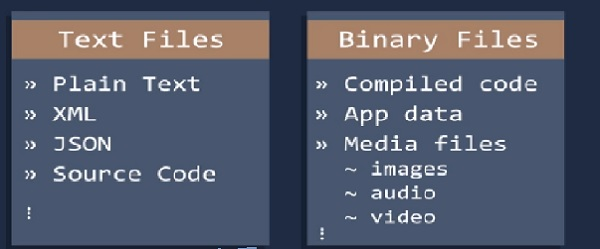# Text Analysis in Python3

PythonProgrammingServer Side Programming

In this assignment we work with files. Files are everywhere in this Universe. In computer system files are essential part. Operating system consists a lot of files.

Python has two types of files-Text Files and Binary Files.Here we discuss about Text Files

Here we focus some of the important functions on files.

• Number of words
• Number of characters
• Average word length
• Number of stop words
• Number of special characters
• Number of numeric
• Number of uppercase words

We have a test file "css3.txt", we are working on that file

## Number of words

When we count number of words in a sentences, we use split function. This is most easiest way. In this case we also apply split function.

## Example code

filename="C:/Users/TP/Desktop/css3.txt"
try:
with open(filename) as file_object:
except FileNotFoundError:
message="sorry" +filename
print(message)
else:
words=contents.split()
number_words=len(words)
print("Total words of" + filename ,"is" , str(number_words))


## Output

Total words of C:/Users/TP/Desktop/css3.txt is 3574


## Number of characters

Here we count the number of characters in a word, here we use the length of the word. If the length is 5 then 5 characters are there in that word.

## Example code

filename="C:/Users/TP/Desktop/css3.txt"
try:
with open(filename) as file_object:
except FileNotFoundError:
message="sorry" +filename
print(message)
else:
words=0
characters=0
wordslist=contents.split()
words+=len(wordslist)
characters += sum(len(word) for word in wordslist)
#print(lineno)
print("TOTAL CHARACTERS IN A TEXT FILE =",characters)


## Output

TOTAL CHARACTERS IN A TEXT FILE = 17783


## Average word length

Here, we calculate the sum of the length of all the words and divide it by the total length.

## Example code

filename="C:/Users/TP/Desktop/css3.txt"
try:
with open(filename) as file_object:
except FileNotFoundError:
message="sorry" +filename
print(message)
else:
words=0
wordslist=contents.split()
words=len(wordslist)
average= sum(len(word) for word in wordslist)/words
print("Average=",average)


## Output

Average= 4.97


## Number of stop words

To solve this we use NLP library in Python.

## Example code

from nltk.corpus import stopwords
from nltk.tokenize import word_tokenize
my_example_sent = "This is a sample sentence"
mystop_words = set(stopwords.words('english'))
my_word_tokens = word_tokenize(my_example_sent)
my_filtered_sentence = [w for w in my_word_tokens if not w in mystop_words]
my_filtered_sentence = []
for w in my_word_tokens:
if w not in mystop_words:
my_filtered_sentence.append(w)
print(my_word_tokens)
print(my_filtered_sentence)


## Number of special characters

Here we can calculating the number of hashtags or mentions present in it. This is helps to extract extra information from our text data.

## Example code

import collections as ct
filename="C:/Users/TP/Desktop/css3.txt"
try:
with open(filename) as file_object:
except FileNotFoundError:
message="sorry" +filename
print(message)
else:
words=contents.split()
number_words=len(words)
special_chars = "#"
new=sum(v for k, v in ct.Counter(words).items() if k in special_chars)
print("Total Special Characters", new)


## Output

Total Special Characters 0


## Number of numeric

Here we can calculate the number of numeric data present in the text files. It is same as the calculation the number of characters in a word.

## Example code

filename="C:/Users/TP/Desktop/css3.txt"
try:
with open(filename) as file_object:
except FileNotFoundError:
message="sorry" +filename
print(message)
else:
words=sum(map(str.isdigit, contents.split()))
print("TOTAL NUMERIC IN A TEXT FILE =",words)


## Output

TOTAL NUMERIC IN A TEXT FILE = 2


## Number of uppercase words

Using isupper() function, we can calculate number of upper case letters in the text.

## Example code

filename="C:/Users/TP/Desktop/css3.txt"
try:
with open(filename) as file_object:
except FileNotFoundError:
message="sorry" +filename
print(message)
else:
words=sum(map(str.isupper, contents.split()))
print("TOTAL UPPERCASE WORDS IN A TEXT FILE =",words)


## Output

TOTAL UPPERCASE WORDS IN A TEXT FILE = 121